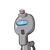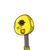# 6. Think of an even number 2x and write algebraic expression fora) The next odd numberb) The next even numberc)

6. Think of an even number 2x and write algebraic expression for
a) The next odd number
b) The next even number
c) The previous even number​

### 2 thoughts on “6. Think of an even number 2x and write algebraic expression for<br />a) The next odd number<br />b) The next even number<br />c)”

1.Step-by-step explanation:

Consider the number sequence 1, 2, 3, 4, 5

here 2,4 are even and 1,3,5 are odd

2x is even by substituting x = Z where Z is an integer

a) To find next odd number, odd number occurs after every number. so, by adding 1 to even number, we can get an odd number.

expression is

### 2x + 1

b) To find next even number, next even number occurs after moving 2 forward from the current even number.

For eg, current even number is 2. next even number is 4 which is obtained by moving 2 steps forward

expression is

### 2x + 2

c) Same as in b) but go 2 steps backward.

expression is

### 2x – 2

2.